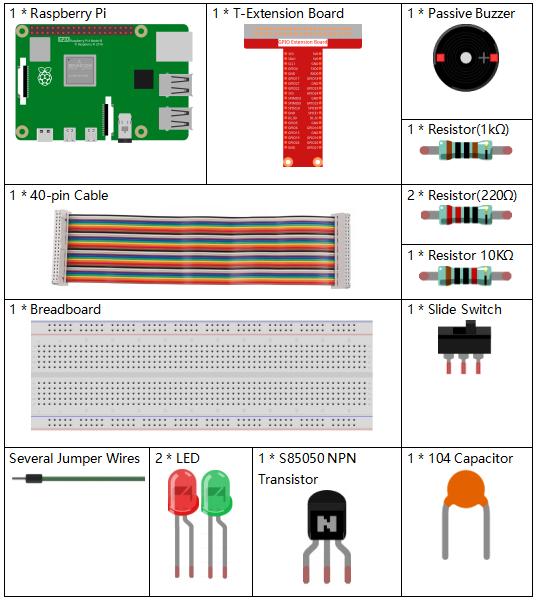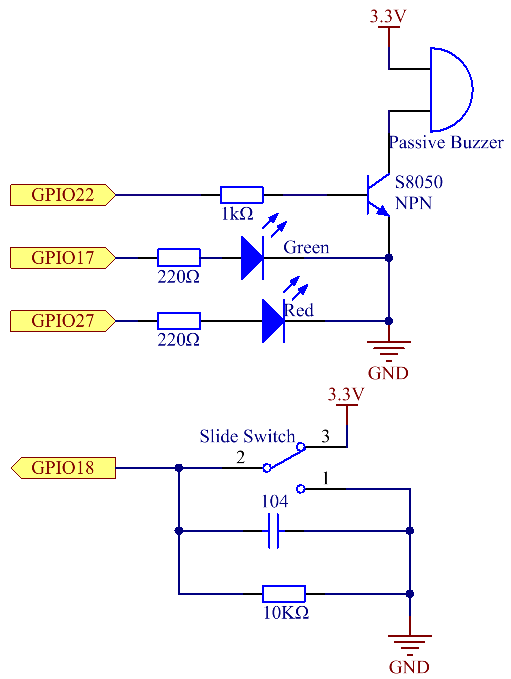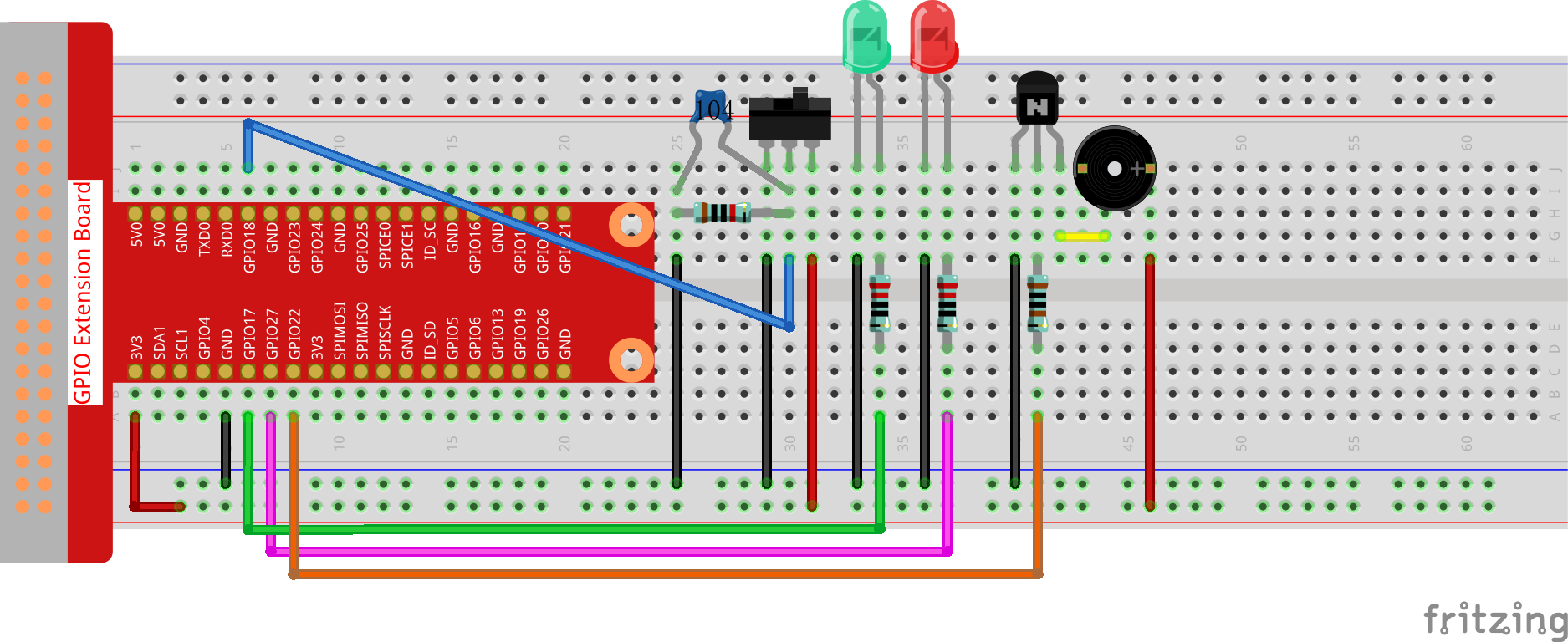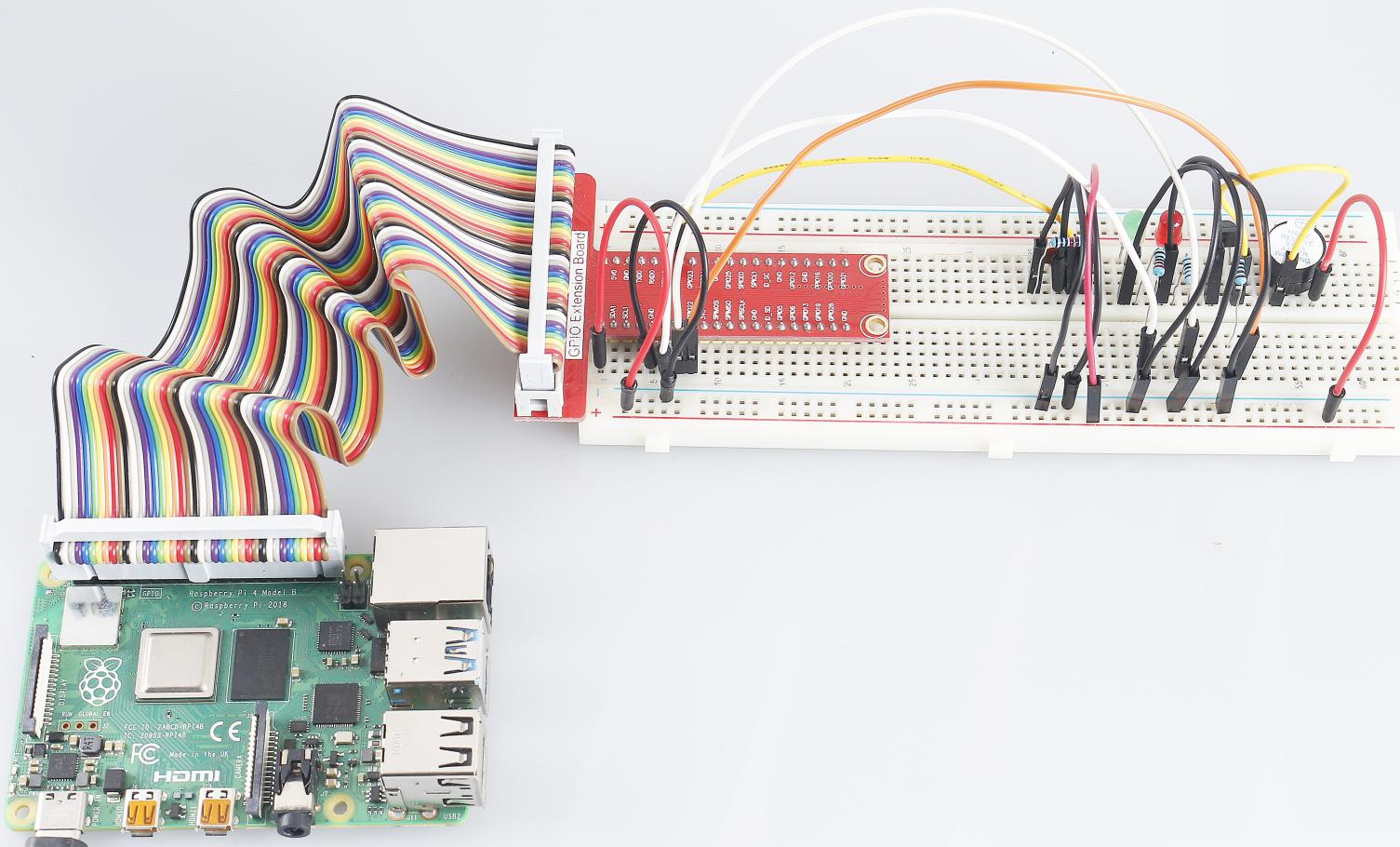# 3.1.10 Alarm Bell¶

## Introduction¶

In this course, we will make a manual alarm device. You can replace the toggle switch with a thermistor or a photosensitive sensor to make a temperature alarm or a light alarm.

## Components¶## Schematic Diagram¶

 T-Board Name physical wiringPi BCM GPIO17 Pin 11 0 17 GPIO18 Pin 12 1 18 GPIO27 Pin 13 2 27 GPIO22 Pin 15 3 22## Experimental Procedures¶

Step 1: Build the circuit.### For C Language Users¶

Step 2: Change directory.

```cd ~/davinci-kit-for-raspberry-pi/c/3.1.10/
```

Step 3: Compile.

```gcc 3.1.10_AlarmBell.c -lwiringPi -lpthread
```

Step 4: Run.

```sudo ./a.out
```

After the program starts, the toggle switch will be toggled to the right, and the buzzer will give out alarm sounds. At the same time, the red and green LEDs will flash at a certain frequency.

Note

If it does not work after running, or there is an error prompt: "wiringPi.h: No such file or directory", please refer to C code is not working?.

Code Explanation

```#include <pthread.h>
```

In this code, you’ll use a new library, pthread.h, which is a set of common thread libraries and can realize multithreading. We add the -lpthread parameter at compile time for the independent working of the LED and the buzzer.

```void *ledWork(void *arg){
while(1)
{
if(flag==0){
}
digitalWrite(ALedPin,HIGH);
delay(500);
digitalWrite(ALedPin,LOW);
digitalWrite(BLedPin,HIGH);
delay(500);
digitalWrite(BLedPin,LOW);
}
}
```

The function ledWork() helps to set the working state of these 2 LEDs: it keeps the green LED lighting up for 0.5s and then turns off; similarly, keeps the red LED lighting up for 0.5s and then turns off.

```void *buzzWork(void *arg){
while(1)
{
if(flag==0){
}
if((note>=800)||(note<=130)){
pitch = -pitch;
}
note=note+pitch;
softToneWrite(BeepPin,note);
delay(10);
}
}
```

The function buzzWork() is used to set the working state of the buzzer. Here we set the frequency as between 130 and 800, to accumulate or decay at an interval of 20.

```void on(){
flag = 1;
if(softToneCreate(BeepPin) == -1){
printf("setup softTone failed !");
return;
}
}
```

In the function on():

1. Define the mark "flag=1", indicating the ending of the control thread.

2. Create a software-controlled tone pin BeepPin.

3. Create two separate threads so that the LED and the buzzer can work at the same time.

Return the Value

If successful, return "0"；otherwise, return the fall number "-1".

Parameter

The first parameter is a pointer to the thread identifier.
The second one is used to set the thread attribute.
The third one is the starting address of the thread running function.
The last one is the one that runs the function.
```void off(){
flag = 0;
softToneStop(BeepPin);
digitalWrite(ALedPin,LOW);
digitalWrite(BLedPin,LOW);
}
```

The function Off() defines "flag=0" so as to exit the threads ledWork and BuzzWork and then turn off the buzzer and the LED.

```int main(){
setup();
int lastState = 0;
while(1){
if ((currentState == 1)&&(lastState==0)){
on();
}
else if((currentState == 0)&&(lastState==1)){
off();
}
lastState=currentState;
}
return 0;
}
```

Main() contains the whole process of the program: firstly read the value of the slide switch; if the toggle switch is toggled to the right (the reading is 1), the function on() is called, the buzzer is driven to emit sounds and the the red and the green LEDs blink. Otherwise, the buzzer and the LED don’t work.

### For Python Language Users¶

Step 2: Change directory.

```cd ~/davinci-kit-for-raspberry-pi/python/
```

Step 3: Run.

```sudo python3 3.1.10_AlarmBell.py
```

After the program starts, the toggle switch will be toggled to the right, and the buzzer will give out alarm sounds. At the same time, the red and green LEDs will flash at a certain frequency.

Code

Note

You can Modify/Reset/Copy/Run/Stop the code below. But before that, you need to go to source code path like `davinci-kit-for-raspberry-pi/python`.

```import RPi.GPIO as GPIO
import time

BeepPin=22
ALedPin=17
BLedPin=27
switchPin=18

Buzz=0
flag =0
note=150
pitch=20

def setup():
GPIO.setmode(GPIO.BCM)
GPIO.setup(BeepPin, GPIO.OUT)
GPIO.setup(ALedPin,GPIO.OUT,initial=GPIO.LOW)
GPIO.setup(BLedPin,GPIO.OUT,initial=GPIO.LOW)
GPIO.setup(switchPin,GPIO.IN)
global Buzz
Buzz=GPIO.PWM(BeepPin,note)

def ledWork():
while flag:
GPIO.output(ALedPin,GPIO.HIGH)
time.sleep(0.5)
GPIO.output(ALedPin,GPIO.LOW)
GPIO.output(BLedPin,GPIO.HIGH)
time.sleep(0.5)
GPIO.output(BLedPin,GPIO.LOW)

def buzzerWork():
global pitch
global note
while flag:
if note >= 800 or note <=130:
pitch = -pitch
note = note + pitch
Buzz.ChangeFrequency(note)
time.sleep(0.01)

def on():
global flag
flag = 1
Buzz.start(50)
tBuzz.start()
tLed.start()

def off():
global flag
flag = 0
Buzz.stop()
GPIO.output(ALedPin,GPIO.LOW)
GPIO.output(BLedPin,GPIO.LOW)

def main():
lastState=0
while True:
currentState =GPIO.input(switchPin)
if currentState == 1 and lastState == 0:
on()
elif currentState == 0 and lastState == 1:
off()
lastState=currentState

def destroy():
off()
GPIO.cleanup()

if __name__ == '__main__':
setup()
try:
main()
except KeyboardInterrupt:
destroy()
```

Code Explanation

```import threading
```

Here, we import the Threading module and it allows you to do multiple things at once, while normal programs can only execute code from top to bottom. With Threading modules, the LED and the buzzer can work separately.

```def ledWork():
while flag:
GPIO.output(ALedPin,GPIO.HIGH)
time.sleep(0.5)
GPIO.output(ALedPin,GPIO.LOW)
GPIO.output(BLedPin,GPIO.HIGH)
time.sleep(0.5)
GPIO.output(BLedPin,GPIO.LOW)
```

The function ledWork() helps to set the working state of these 2 LEDs: it keeps the green LED lighting up for 0.5s and then turns off; similarly, keeps the red LED lighting up for 0.5s and then turns off.

```def buzzerWork():
global pitch
global note
while flag:
if note >= 800 or note <=130:
pitch = -pitch
note = note + pitch
Buzz.ChangeFrequency(note)
time.sleep(0.01)
```

The function buzzWork() is used to set the working state of the buzzer. Here we set the frequency as between 130 and 800, to accumulate or decay at an interval of 20.

```def on():
global flag
flag = 1
Buzz.start(50)
tBuzz.start()
tLed.start()
```

In the function on():

1. Define the mark "flag=1", indicating the ending of the control thread.

2. Start the Buzz, and set the duty cycle to 50%.

3. Create 2 separate threads so that the LED and the buzzer can work at the same time.

Among the construction methods, the principal parameter is target, we need to assign a callable object (here are the functions ledWork and BuzzWork ) to target.

Next start() is called to start the thread object, ex., tBuzz.start() is used to start the newly installed tBuzz thread.

```def off():
global flag
flag = 0
Buzz.stop()
GPIO.output(ALedPin,GPIO.LOW)
GPIO.output(BLedPin,GPIO.LOW)
```

The function Off() defines "flag=0" so as to exit the threads ledWork and BuzzWork and then turn off the buzzer and the LED.

```def main():
lastState=0
while True:
currentState =GPIO.input(switchPin)
if currentState == 1 and lastState == 0:
on()
elif currentState == 0 and lastState == 1:
off()
lastState=currentState
```

Main() contains the whole process of the program: firstly read the value of the slide switch; if the toggle switch is toggled to the right (the reading is 1), the function on() is called, the buzzer is driven to emit sounds and the the red and the green LEDs blink. Otherwise, the buzzer and the LED don’t work.

## Phenomenon Picture¶